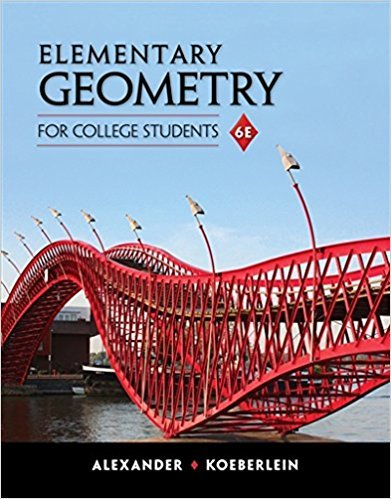×
×

# In Exercises 11 to 22, sketch and describe each locus in the plane. Find the locus ofISBN: 9781285195698 295

## Solution for problem 15 Chapter 7

Elementary Geometry for College Students | 6th Edition

• Textbook Solutions
• 2901 Step-by-step solutions solved by professors and subject experts
• Get 24/7 help from StudySoup virtual teaching assistantsElementary Geometry for College Students | 6th Edition

4 5 0 365 Reviews
27
4
Problem 15

In Exercises 11 to 22, sketch and describe each locus in the plane. Find the locus of points that are equidistant from three noncollinear points D, E, and F.

Step-by-Step Solution:
Step 1 of 3

Calculus 3 EX . Show that the equation ×2+y2+ -22-6×22=11 . 4y a sphere . Find center and radius if so . we around gives want move = ( X -a)2 + which...

Step 2 of 3

Step 3 of 3

##### ISBN: 9781285195698

Unlock Textbook Solution